KNOWLEDGEBASE - ARTICLE #2212

# Bug Fixed: User-defined equations containing fractions of same-base exponents evaluated incorrectly

## The big picture

With the release of Prism 9.2.0, an issue was fixed with the way some user-defined equations were simplified for evaluation. Prior to this fix, some user-defined equations containing fractions of exponents with the same base were simplified incorrectly, resulting in missing results. This issue affected earlier versions of Prism 9 (but not Prism 8), and has been corrected in Prism 9.2.0. However, the curves generated as a result of this issue would have been almost immediately wrong. It's unlikely that you would have experienced this issue without noticing it. That said, these user-defined equations should once again work as expected. Additionally, this issue had no effect on any of the existing built-in equations offered in Prism's equation library, and we've added additional test cases to ensure that similar issues will be detected in the future.

## The details

Prism's equation evaluation engine includes a set of rules that it uses to simplify user-defined equations based on basic properties of mathematical operations and functions. For example: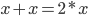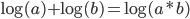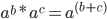The issue that was fixed in Prism 9.2.0 involved updating the following incorrect rule definition: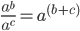to the mathematically correct definition: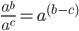Note that only user-defined equations that utilized this form of same-base exponent fraction would have been affected. If the user-defined equation included the simplified a^(b-c) version initially, Prism would have properly evaluated this expression in both 9.2.0 and previous versions.

## Explore the Knowledgebase

Analyze, graph and present your scientific work easily with GraphPad Prism. No coding required.